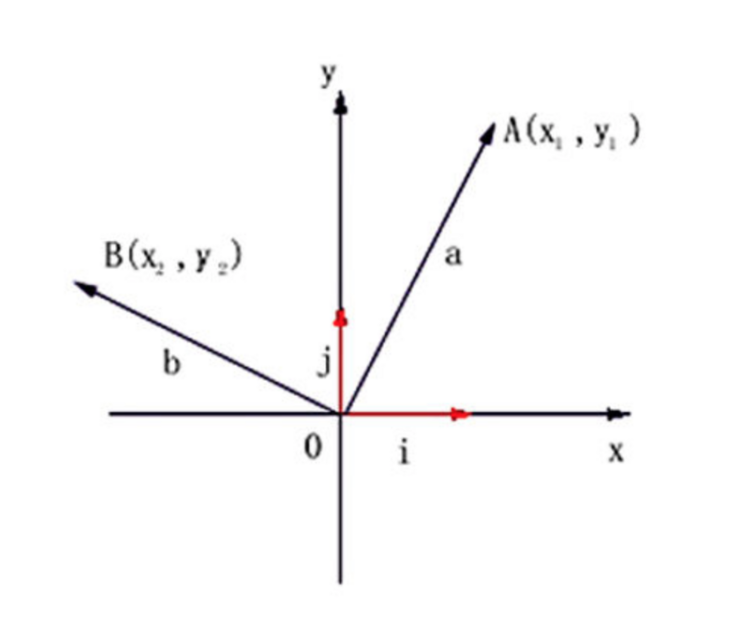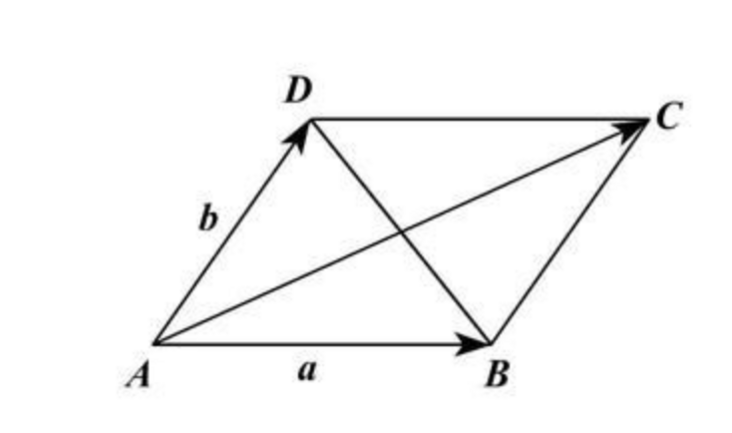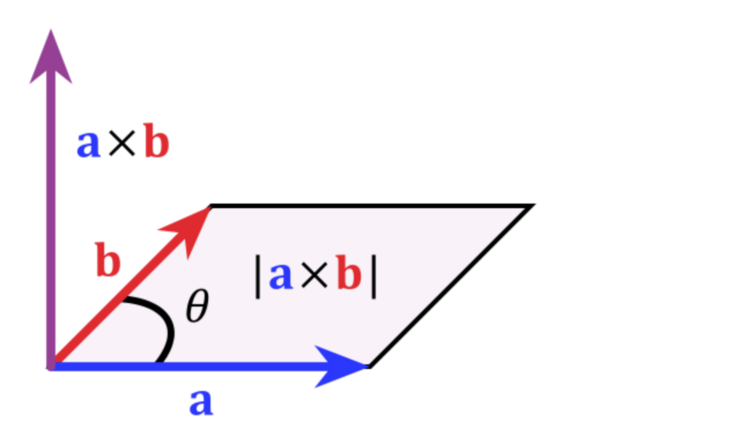• 结构-03. 平面向量加法(10) java
2021-03-09 07:45:14

本题要求编写程序，计算两个二维平面向量的和向量。

输入格式：

输入在一行中按照“x1 y1 x2 y2”的格式给出两个二维平面向量V1=(x1, y1)和V2=(x2, y2)的分量。

输出格式：

在一行中按照“(x, y)”的格式输出和向量，坐标输出小数点后1位(注意不能输出-0.0)。

输入样例：

3.5 -2.7 -13.9 8.7

输出样例：

(-10.4, 6.0)

import java.util.*;

import static java.lang.System.*;

class Struct{

double x,y;

public Struct(double num1,double num2){

x=num1;

y=num2;

}

void printf(){

out.printf("(%.1f, %.1f)",x,y);

out.println();

}

double x3=x+e2.x,y3=y+e2.y;

if(x3>-0.05&&x3<0)//比如 －0.03，因为只显示一位小数，因此会输出－0.0，所以只要将这类数 改成0 即可

x3=0.01;

if(y3>-0.05&&y3<0)

y3=0;

Struct e3=new Struct(x3,y3);

return e3;

}

}

public class Main {

public static void main(String [] args){

Scanner scan=new Scanner(in);

while(scan.hasNext()){

double x1,y1,x2,y2;

x1=scan.nextDouble();

y1=scan.nextDouble();

x2=scan.nextDouble();

y2=scan.nextDouble();

Struct e1,e2;

e1=new Struct(x1,y1);

e2=new Struct(x2,y2);

e3.printf();

}

}

}

更多相关内容
• 平面向量的计算和运用实例相关概念有向线段：具有方向的线段叫做有向线段，以A为起点，B为终点的有向线段记作 或AB；向量的模：有向线段AB的长度叫做向量的模，记作|AB|；零向量：长度等于0的向量叫做零向量，记作 ...

平面向量的计算和运用实例

相关概念

有向线段：具有方向的线段叫做有向线段，以A为起点，B为终点的有向线段记作 或AB；

向量的模：有向线段AB的长度叫做向量的模，记作|AB|；

零向量：长度等于0的向量叫做零向量，记作 或0。(注意粗体格式，实数“0”和向量“0”是有区别的，书写时要在向量“0”上加箭头，以免混淆)；

相等向量：长度相等且方向相同的向量叫做相等向量；

平行向量(共线向量)：两个方向相同或相反的非零向量叫做平行向量或共线向量，-零向量与任意向量平行，即0//a；

单位向量：模等于1个单位长度的向量叫做单位向量，通常用e表示，平行于坐标轴的单位向量习惯上分别用i、j表示。

相反向量：与a长度相等，方向相反的向量，叫做a的相反向量，-(-a)=a，零向量的相反向量仍然是零向量。 

几何表示二维

任取平面上两点A(x1,y1),B(x2,y2),则向量AB=(x2-x1,y2-y1)，即一个向量的坐标等于表示此向量的有向线段的终点坐标减去始点的坐标。

三维

A(x1,y1,z1),B(x2,y2,z2),向量AB=(x2-x1,y2-y1,z2-z1)

基本计算

向量相加

二维

平行四边形定则解决向量加法的方法：将两个向量平移至公共起点，以向量的两条边作平行四边形，向量的加法结果为公共起点的对角线。向量的点乘:a * b

公式：a b = |a| |b| * cosθ

点乘又叫向量的内积、数量积，是一个向量和它在另一个向量上的投影的长度的乘积；是标量。

点乘反映着两个向量的“相似度”，两个向量越“相似”，它们的点乘越大。

向量的叉乘：a ∧ b

方向：a向量与b向量的向量积的方向与这两个向量所在平面垂直，且遵守右手定则。

右手定则：右手的四指方向指向第一个矢量,屈向叉乘矢量的夹角方向(两个矢量夹角方向取小于180°的方向),那么此时大拇指方向就是叉乘所得的新的矢量的方向.(大拇指应与食指成九十度)

在二维中，两个向量的向量积的模的绝对值等于由这两天向量组成的平行四边形的面积二维

(x1,y1)X(x2,y2)=(x1y2-x2y1)

三维

(x1,y1,z1)X(x2,y2,z2)=(y1z2-y2z1, z1x2-z2y1, x1y2-x2y1)

实例运用

//计算向量叉乘

var crossMul=function(v1,v2){

return v1.x*v2.y-v1.y*v2.x;

}

//javascript判断两条线段是否相交

var checkCross=function(p1,p2,p3,p4){

var v1={x:p1.x-p3.x,y:p1.y-p3.y};

v2={x:p2.x-p3.x,y:p2.y-p3.y};

v3={x:p4.x-p3.x,y:p4.y-p3.y};

v=crossMul(v1,v3)*crossMul(v2,v3);

v1={x:p3.x-p1.x,y:p3.y-p1.y};

v2={x:p4.x-p1.x,y:p4.y-p1.y};

v3={x:p2.x-p1.x,y:p2.y-p1.y};

return (v<=0&&crossMul(v1,v3)*crossMul(v2,v3)<=0)?true:false;

}

//判断点是否在多边形内

var checkPP=function(point,polygon){

var p1,p2,p3,p4;

p1=point;

p2={x:-100,y:point.y};

var count=0;

//对每条边都和射线作对比

for(var i=0;i

p3=polygon[i];

p4=polygon[i+1];

if(checkCross(p1,p2,p3,p4)==true){

count++;

}

}

p3=polygon[polygon.length-1];

p4=polygon;

if(checkCross(p1,p2,p3,p4)==true){

count++;

}

// console.log(count)

return (count%2==0)?false:true;

}

展开全文• Java实现的平面向量基本运算，其中涉及到了有关数学方面的角度、弧度转换的基本问题，记录一下，感觉大学学的线性代数都忘记的差不多了=.=下面是一个Vector2D的向量类，里面封装了一些关于向量的基本运算的函数。...

Java实现的平面向量基本运算，其中涉及到了有关数学方面的角度、弧度转换的基本问题，记录一下，感觉大学学的线性代数都忘记的差不多了=.=

下面是一个Vector2D的向量类，里面封装了一些关于向量的基本运算的函数。

//平面向量(x,y)的基本运算规则,角度弧度的转换等实现

public class Vector2D {

private double x;

private double y;

public Vector2D()

{

x = 0;

y = 0;

}

public Vector2D(double _x, double _y)

{

x = _x;

y = _y;

}

//获取弧度

{

return Math.atan2(y, x);

}

//获取角度

public double getAngle()

{

return getRadian() / Math.PI * 180;

}

public Vector2D clone()

{

return new Vector2D(x,y);

}

public double getLength()

{

return Math.sqrt(getLengthSQ());

}

public double getLengthSQ()

{

return x * x + y * y;

}

//向量置零

public Vector2D Zero()

{

x = 0;

y = 0;

return this;

}

public boolean isZero()

{

return x == 0 && y == 0;

}

//向量的长度设置为我们期待的value

public void setLength(double value)

{

double _angle = getAngle();

x = Math.cos(_angle) * value;

y = Math.sin(_angle) * value;

}

//向量的标准化(方向不变，长度为1)

public Vector2D normalize()

{

double length = getLength();

x = x / length;

y = y / length;

return this;

}

//是否已经标准化

public boolean isNormalized()

{

return getLength() == 1.0;

}

//向量的方向翻转

public Vector2D reverse()

{

x = -x;

y = -y;

return this;

}

//2个向量的数量积(点积)

public double dotProduct(Vector2D v)

{

return x * v.x + y * v.y;

}

//2个向量的向量积(叉积)

public double crossProduct(Vector2D v)

{

return x * v.y - y * v.x;

}

//计算2个向量的夹角弧度

//参考点积公式:v1 * v2 = cos * |v1| *|v2|

public static double radianBetween(Vector2D v1, Vector2D v2)

{

if(!v1.isNormalized()) v1 = v1.clone().normalize(); // |v1| = 1

if(!v2.isNormalized()) v2 = v2.clone().normalize(); // |v2| = 1

return Math.acos(v1.dotProduct(v2));

}

//弧度 = 角度乘以PI后再除以180、 推理可得弧度换算角度的公式

//弧度转角度

{

return radian / Math.PI * 180;

}

//向量加

{

return new Vector2D(x + v.x, y + v.y);

}

//向量减

public Vector2D subtract(Vector2D v)

{

return new Vector2D(x - v.x, y - v.y);

}

//向量乘

public Vector2D multiply(double value)

{

return new Vector2D(x * value, y * value);

}

//向量除

public Vector2D divide(double value)

{

return new Vector2D(x / value, y / value);

}

}

展开全文• Java实现的二维向量类，包含向量的所有基本运算。可以表示平面中的一个点或一个向量
• Java自定义二维向量，实现向量之间，加减乘除，取角度等操作 /** 自定义，平面二维向量类 **/ public class Vector2D implements Cloneable { private double x; private double y; //get public double getX...

Java自定义二维向量，实现向量之间，加减乘除，取角度等操作


/**  自定义，平面二维向量类  **/
public class Vector2D implements Cloneable {
private double x;
private double y;

//get
public double getX() { return x;}
public double getY() { return y;}

//set
public void setX(double x) { this.x = x;}
public void setY(double y) { this.y = y;}

@Override
public String toString() {
return "Vector2D{" +
"x=" + x +
", y=" + y +
'}';
}

/** 空构造 默认值V(x,y)=>V(0,0) **/
public Vector2D()
{
x = 0;
y = 0;
}

/** 赋值构造 V(x,y) **/
public Vector2D(double _x, double _y)
{
x = _x;
y = _y;
}

/**  获取弧度  **/
{
return Math.atan2(y, x);
}

/**  获取角度  **/
public double getAngle()
{
return getRadian() / Math.PI * 180;
}

/**  克隆该对象  **/
@Override
public Vector2D clone()
{
return new Vector2D(x,y);
}

public double getLength()
{
return Math.sqrt(getLengthSQ());
}

public double getLengthSQ()
{
return x * x + y * y;
}

/**  向量置零  **/
public Vector2D Zero()
{
x = 0;
y = 0;
return this;
}

/** 该向量是否是置零的，是返回true，反之false **/
public boolean isZero()
{
return x == 0 && y == 0;
}

/** 向量的长度设置为我们期待的value  **/
public void setLength(double value)
{
double _angle = getAngle();
x = Math.cos(_angle) * value;
y = Math.sin(_angle) * value;
}

/**  向量的标准化（方向不变，长度为1）  **/
public Vector2D normalize()
{
double length = getLength();
x = x / length;
y = y / length;
return this;
}

/**  是否已经标准化  **/
public boolean isNormalized()
{
return getLength() == 1.0;
}

/**  向量的方向翻转  **/
public Vector2D reverse()
{
x = -x;
y = -y;
return this;
}

/**  2个向量的数量积(点积)  **/
public double dotProduct(Vector2D v)
{
return x * v.x + y * v.y;
}

/**  2个向量的向量积(叉积)  **/
public double crossProduct(Vector2D v)
{
return x * v.y - y * v.x;
}

/**  计算2个向量的夹角弧度  **/
/**  参考点积公式:v1 * v2 = cos<v1,v2> * |v1| *|v2|  **/
public static double radianBetween(Vector2D v1, Vector2D v2)
{
if(!v1.isNormalized()) {
v1 = v1.clone().normalize(); // |v1| = 1
}
if(!v2.isNormalized()) {
v2 = v2.clone().normalize(); // |v2| = 1
}
return Math.acos(v1.dotProduct(v2));
}

//弧度 = 角度乘以PI后再除以180、 推理可得弧度换算角度的公式
/**  弧度转角度  **/
{
return radian / Math.PI * 180;
}

/** 向量加 **/
{
return new Vector2D(x + v.x, y + v.y);
}

/** 向量减 **/
public Vector2D subtract(Vector2D v)
{
return new Vector2D(x - v.x, y - v.y);
}

/** 向量乘 **/
public Vector2D multiply(double value)
{
return new Vector2D(x * value, y * value);
}

/** 向量除 **/
public Vector2D divide(double value)
{
return new Vector2D(x / value, y / value);
}
}

展开全文• 7-65 平面向量加法（15 分） 本题要求编写程序，计算两个二维平面向量的和向量。 输入格式: 输入在一行中按照“x​1​​ y​1​​ x​2​​ y​2​​”的格式给出两个二维平面向量v​1​​=(x​1​​,y​1​​...
• 作者|Anuj Shrivastav 编译|VK 来源|Medium 介绍监督学习...我们将在此博客中讨论的一种这样的模型是支持向量机，简称为SVM。我的目的是为你提供简单明了的SVM内部工作。假设我们正在处理二分类任务。 可能有无限...
• 习题9-3 平面向量加法 在做这个题目的时候，没有考虑到当结果大于-0.05但仍为负数时候，会出现-0.0这样的情况 只有绝对值大于0.05，四舍五入是0.1，就没负数的情况 所以当绝对值小于0.05的时候，直接处理成0. 但是我...c语言
• 以多维几何空间考虑，两组向量的相似度可以描述为在多维几何空间中的距离关系，距离越远，相似度越低。对原文有修改，如有疑惑，请拜访原文。在做分类时常常需要估算不同样本之间的相似性度量(Similarity ...
• 对两个向量执行点乘运算，就是对这两个向量对应位一一相乘之后求和的操作，如下所示，对于向量a和向量b： a和b的点积公式为：这里要求一维向量a和向量b的行列数相同。注意：点乘的结果是一个标量(数量而不是向量)...
• 上一篇文章我们介绍了使用逻辑回归来处理分类问题，本文我们讲一个更强大的分类模型。本文依旧侧重代码实践，你...一、什么是支持向量机SMV在众多实例中寻找一个最优的决策边界，这个边界上的实例叫做支持向量，它们...
• 一、向量的内积1.1向量内积的定义概括地说，向量的内积(点乘/点积/数量积)就是对两个向量执行点乘运算，即对这两个向量对应位一一相乘之后求和的操作，如下所示，对于向量a和向量b： a和b的点积公式为：这里要求一维...
• 一、向量检索图解总结 原文： 大规模特征向量检索算法总结 (LSH PQ HNSW)：https://www.6aiq.com/article/1587522027341 「向量召回」相似检索算法——HNSW：https://mp.weixin.qq.com/s/dfdNj9CZ3Kj2UwDr9PQcVg 二...哈希算法 算法 PQ
• 习题9-3 平面向量加法 本题要求编写程序，计算两个二维平面向量的和向量。 输入格式: 输入在一行中按照“x1 y1 x​2 y​2 ​​ ”的格式给出两个二维平面向量v1=(x1, y1) 和v2=(x2 ,y2) 的分量。 输出格式: 在一行中...c++ 算法 数据结构 c语言 程序设计
• 今天学习了一下《计算几何》，里面讲了一下关于判断一个点是否在某个三角形内的问题(在二维平面上)。其中有一个算法是“同向法”，主要是用...图1好了，言归正传，我们只知道在二维平面中，两个向量的叉乘其结果(...
• 四、Java通过SimHash计算文本内容相似度代码示例 一）、新增依赖包 二）、过滤特殊字符 三）、计算单个分词的Hash值 四）、分词计算向量 五）、获取标题内容的海明距离 六）、获取标题内容的相似度 七）、...标题相似度计算 SimHash
• 概述线性回归是机器学习的最基本算法，本文向读者介绍了线性回归的原理和使用，以及如何通过Java语言编写线性回归的模型代码。并且以水泥质量预测为例构造出一个水泥质量预测模型。还通过线性回归介绍了机器学习的...
• SVM算法(Support Vector Machine，支持向量机)的核心思想有2点：1、如果数据线性可分，那么基于最大间隔的方式来确定超平面，以确保全局最优，使得分类器尽可能健壮；2、如果数据线性不可分，通过核函数将低维样本...
• 平面向量加法(10) 时间限制 400 ms 内存限制 65536 kB 代码长度限制 8000 B 判题程序 Standard 作者 乔林（清华大学） 本题要求编写程序，计算两...ACM
• 1、一、平面的点法式方程,二、平面的一般方程,平面及其方程,一、平面的点法式方程,设一平面通过已知点,且垂直于非零向,称式为平面的点法式方程,求该平面的方程,法向量,量,则有,故,例1.求过三点,即,解: 取该平面 的法...
• 二、支持向量机核心思想 支持向量机实现是通过某种事先选择的非线性映射（核函数）将输入向量映射到一个高维特征空间，在这个空间中构造最优分类超平面。我们使用使用SVM进行数据集分类工作的过程首先是通过预先...
• java 三维向量类 分装了部分向量相关函数 欢迎补充 (提供平面求垂直向量 向量旋转 向量求膜 向量转单位向量等函数)Android
• Time Limit: 3000/1000 MS (Java/Others) Memory Limit: 32768/32768 K (Java/Others) Total Submission(s): 5575 Accepted Submission(s): 2531 Problem Description 话说上回讲到海东集团推选老HDU 高中数学
• 平面直角坐标系xOy中设∠β的始边为x轴的正半轴，设点P（x，y）为∠β的终边上不与原点O重合的任意一点，设r=OP，令∠β=∠α，则： sinα = y / r; cosα = x / r; tanα = y / x; 其实也是通过构建直角...角度计算
• 现在看Direct3D完全没有那时的感觉了，只是矩阵变换的知识是大一下学期学的，现在都忘了，完全不知道诸如求平面的法线之类的方法了。 昨天晚上帮别人写了个程序，距离向量路由 typedef struct { int dis; int from; ......

# java 平面向量java 订阅